# OliNo

Renewable Energy

## Luxerna Downlight D1515 750

Posted by Marcel van der Steen in Led lights, Light measurements Add comments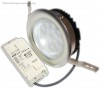presents a led downlighter with a neutral white color temperature. It has a separate power supply to generate the constant current.

This article shows the measurement results. Many parameters are also found in the Eulumdat file.

See this overview for a comparison with other light bulbs.

### Summary measurement data

parameter meas. result remark
Color temperature 5032 K Neutral white (to cold white)
Luminous intensity Iv 1525 Cd Measured straight underneath the lamp
Beam angle 51 deg Valid for all C-angles as there is symmetry along the 1st axis.
Power P 19.3 W
Power Factor 0.95 For every 1 kWh net power consumed, there has been 0.3 kVAhr for reactive power.
Luminous flux 1498 Lm
Luminous efficacy 77 Lm/W
CRI_Ra 68 Color Rendering Index.
Coordinates chromaticity diagram x=0.3448 and y=0.3521
Fitting 230V It is a downligher used with a separate current source.
PAR-value 13.2 μMol/s/m2 The number of photons seen by an average plant when it is lit by the light of this light bulb. Value valid at 1 m distance from light bulb.
PAR-photon efficacy 0.7 μMol/s/We The toal emitted number of photons by this light, divided by its consumption in W. It indicates a kind of efficacy in generating photons.
S/P ratio 1.7 This factor indicates the amount of times more efficient the light of this light bulb is perceived under scotopic circumstances (ow environmental light level).
D x H external dimensions 168 x 58 mm External dimensions of the lamp (D = diameter).
D luminous area 92 mm Dimensions of the luminous area (used in Eulumdat file). This is equal to the surface of the glass on the front.
form factor recessed
General remarks The ambient temperature during the whole set of measurements was 24-26.5 deg C. The maximum temperature of the tube light gets about 24 degrees hotter than ambient.
Warm up effect: during the warm up time the variations in illuminance and consumed power are less than 5 %.Voltage dependency: the power consumption and illuminance do noy depend on the voltage when it is varied from 200 – 250 V.
Measurement report (PDF)Eulumdat fileRight click on icon and save the file.

### Overview table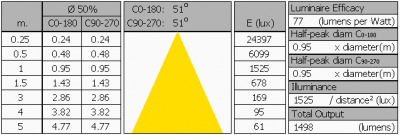The overview table is explained on the OliNo website. Please note that this overview table makes use of calculations, use this data with care as explained on the OliNo site. E (lux) values are not accurate, when within 5 x 92 mm = 460 mm. Within this distance from the lamp, the measured lux values willl be less than the computed values in this overview as the measurements are then within the near field of the lamp.

### Eulumdat light diagram

This light diagram below comes from the program Qlumedit, that extracts these diagrams from an Eulumdat file. It is explained on the OliNo site.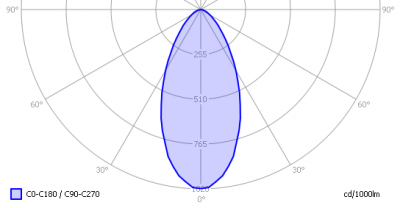The light diagram giving the radiation pattern.

It indicates the luminous intensity around the light bulb. The directions C90-C270 and C0-C180 give the same result as there is a symmetry along the 1st axis.

### Illuminance Ev at 1 m distance, or luminous intensity Iv

Herewith the plot of the averaged luminous intensity Iv as a function of the inclination angle with the light bulb.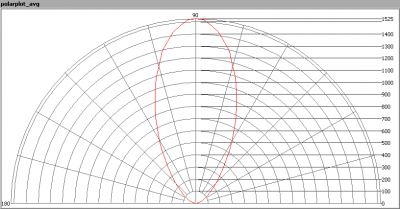The radiation pattern of the light bulb.

This radiation pattern is the average of the light output of the light diagram given earlier. Also, in this graph the luminous intensity is given in Cd.

These averaged values are used (later) to compute the lumen output.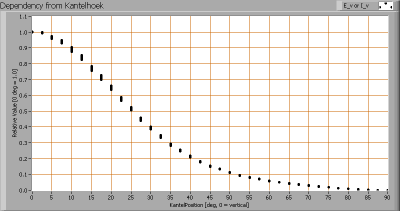Intensity data of every measured turn angle at each inclination angle.

This plot shows per inclination angle the intensity measurement results for each turn angle at that inclination angle. There normally are differences in illuminance values for different turn angles. However for further calculations the averaged values will be used.

When using the average values per inclination angle, the beam angle can be computed, being 51º.

### Luminous flux

With the averaged illuminance data at 1 m distance, taken from the graph showing the averaged radiation pattern, it is possible to compute the luminous flux.

The result of this computation for this light spot is a luminous flux of 1498 Lm.

### Luminous efficacy

The luminous flux being 1498 Lm, and the power of the light bulb being 19.3 W, yields a luminous efficacy of 77 Lm/W.

A power factor of 0.95 means that for every 1 kWh net power consumed, a reactive component of 0.3 kVAr was needed.

 Lamp voltage 230 VAC Lamp current 88 mA Power P 19.3 W Apparent power S 20.3 VA Power factor 0.95

Of this light bulb the voltage across ad the resulting current through it are measured and graphed. See the OliNo site how this is obtained.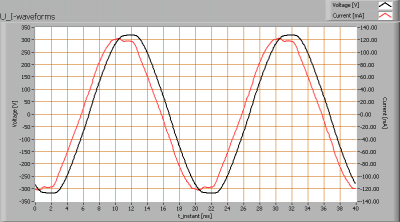Voltage across and current through the lightbulb

This waveforms have been checked on requirements posed by the norm IEC 61000-3-2:2006 (including up to A2:2009). See also the explanation on the OliNo website.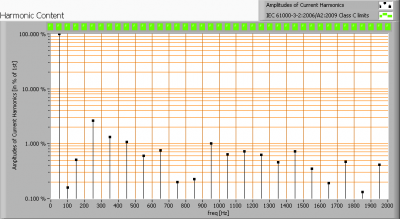Harmonics in in the current waveform and checked against IEC61000-3-2:2006

There are no limits defined in the IEC norm for led lighting equipment with P <= 25 W.

The Total Harmonic Distortion of the current is computed as 4 %.

### Temperature measurements lamp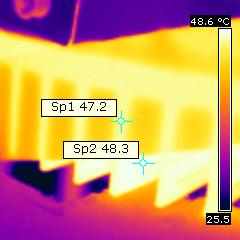Temperature image of the light bulb, temperature probed on heat sink

The tape is not needed; the temperature of the side of the heat sink ribs is the same as where no tape is applied.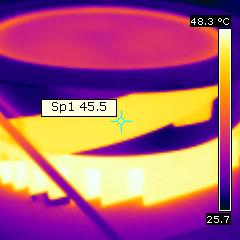Temperature image of the light bulb, temperature probed at side cover

Tape is put on the housing and clearly visible on the second image where the external housing is measured.

 status lamp > 2 hours on ambient temperature 24 deg C reflected background temperature 24 deg C camera Flir B-CAM Western S emissivity 0.95(1) measurement distance 0.10 and 0.30 m IFOVgeometric 0.4 and 1.1 mm NETD (thermal sensitivity) 100 mK

(1) The emissivity of the masking tape is used which is about 0.95. The masking tape is so thin that it quickly takes the same temperature as the light bulb’s heat sink.

The lamp on its hotttest place (approximately 48 degrees) gets a bit warmer than warm-to-the-touch.

### Color temperature and Spectral power distribution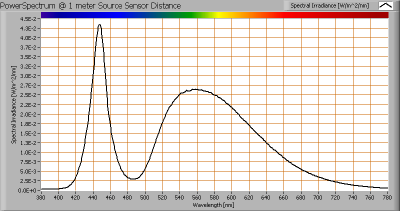The spectral power distribution of this light bulb, energies on y-axis valid at 1 m distance.

The measured color temperature is about 5025 K which is neutral white.

This color temperature is measured straight underneath the light bulb. Below a graph showing the color temperature for different inclination angles.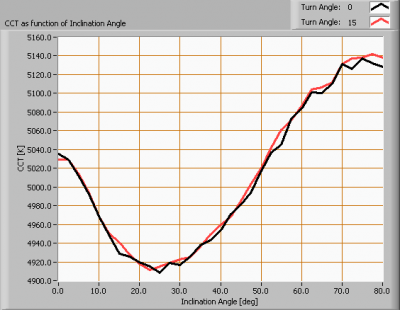Color temperature as a function of inclination angle.

The measurement of CCT is measured for inclination angles up to 80º since then the illuminance value was decreased to very low values (< 5 lux).

The beam angle is maximally 51º, meaning a 25.5º inclination angle. In this area the majority of the light is present. The variation in correlated color temperature in this area is about 2 %.

### PAR value and PAR spectrum

To make a statement how well the light of this light bulb is for growing plants, the PAR-area needs to be determined. See the OliNo website how this all is determined and the explanation of the graph.The photon spectrum, then the sensitivity curve and as result the final PAR spectrum of the light of this light bulb

parameter value unit
PAR-number 13.2 μMol/s/m²
PAR-photon current 13.0 μMol/s
PAR-photon efficacy 0.7 μMol/s/W

The PAR efficiency is 64 % (valid for the PAR wave length range of 400 – 700 nm). So maximally 64 % of the total of photons in the light is effectively used by the average plant (since the plant might not take 100 % of the photons at the frequency where its relative sensitivity is 100 %).

### S/P ratio

The S/P ratio and measurement is explained on the OliNo website. Here the results are given.The power spectrum, sensitivity curves and resulting scotopic and photopic spectra (spectra energy content defined at 1 m distance).

The S/P ratio is 1.7.

More info on S/P ratio can be found on the OliNo website.

### Chromaticity diagram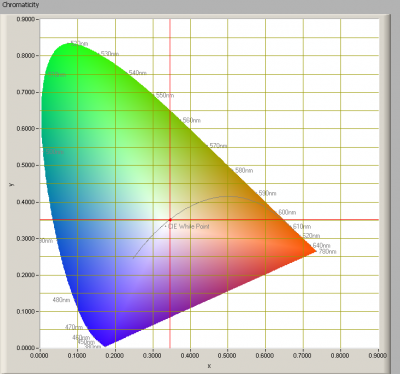The chromaticity space and the position of the lamp’s color coordinates in it.

The light coming from this lamp is on top of the Planckian Locus (the black path in the graph).

Its coordinates are x=0.3448 and y=0.3521.

### Color Rendering Index (CRI) or also Ra

Herewith the image showing the CRI as well as how well different colors are represented (rendered). The higher the number, the better the resemblance with the color when a black body radiator would have been used (the sun, or an incandescent lamp). Practical information and also some critics about the CRI can be found on the OliNo website.

Each color has an index Rx, and the first 8 indexes (R1 .. R8) are averaged to compute the Ra which is equivalent to the CRI.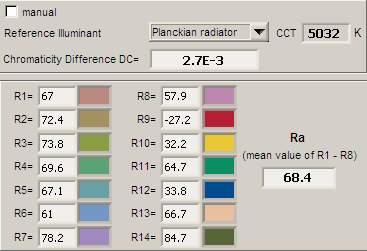CRI of the light of this lightbulb.

The value of 68 is lower than 80 which is considered a minimum value for indoor usage.

Note: the chromaticity difference is 0.0027 indicates the distance to the Planckian Locus. Its value is lower than 0.0054, which means that the calculated CRI result is meaningful.

### Voltage dependency

The dependency of a number of lamp parameters on the lamp voltage is determined. For this, the lamp voltage has been varied and its effect on the following light bulb parameters measured: illuminance E_v [lx], the lamppower P [W], the (Correlated) Color Temperature [K] and the luminous efficacy [Lm/W].Lamp voltage dependencies of certain light bulb parameters, where the value at 230 V is taken as 100 %.

The illuminance and consumed power do not significantly vary when the voltage is varied.

When the voltage at 230 V varies with + and – 5 V, then the illuminance varies < 0.1 %, so when abrupt voltage changes occur this effect is not visible in the illuminance output.

### Warm up effects

After switch on of a cold lamp, the effect of heating up of the lamp is measured on illuminance E_v [lx], color temperature CT or correlated color temperature CCT [K], the lamppower P [W] and the luminous efficacy [lm/W].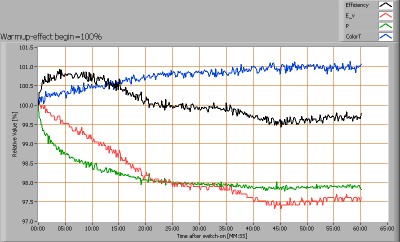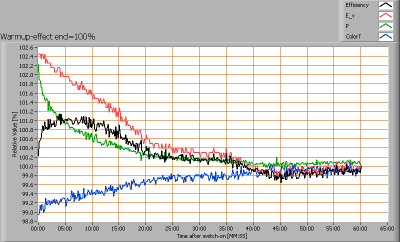Effect of warming up on different light bulb parameters. At top the 100 % level is put at begin, and at bottom at the end.

The warm up time is about 40 minutes. During that time the variations in illuminance and consumed power are less than 5 %.